# RHRT: Finding Premature Ventricular Complexes

#### 2021-06-28

The RHRT package helps you assess Heart Rate Turbulence (HRT) in RR intervals and calculate turbulence onset (TO), slope (TS) and timing (TT). It can plot the tachograms and checks the results for reliability. The ventricular premature beats (VPCs) with coupling (CPI) and compensatory interval (CMI) can either be given with annotations or found on the basis of the filter rules as first described by Grimm et al. 2003. The type of average and order of calculation for all parameters can be set.

## Synopsis for the hasty (Quick-start guide)

This chapter sums up the most common functions and parameters needed when using RHRT.

library("RHRT")
# testdataLong is a numeric vector of RR intervals in msec
data("testdataLong", package = "RHRT")
ints <- testdataLong
# testdataLong_Ann is a character vector of annotations corresponding to testdataLong
data("testdataLong_Ann", package = "RHRT")
ann <- testdataLong_Ann

### Checking interval data for HRTs

The core function of RHRT is vectorToHRT that finds valid VPCs in RR intervals and returns an HRTList object (see chapter HRTList object for more information):

hrtl <- vectorToHRT(ints) 

Every RR interval sequence that matches the needed interval lengths is considered to be a coupling and compensatory interval of a VPC, which can lead to wrong matches. If your data is annotated, you can provide the annotation data with the parameters annotations and PVCAnn.

hrtl <- vectorToHRT(ints, annotations = ann, PVCAnn = "V")

Other parameters are:

• numPreRRs & numPostRRs are used to modify the filter rules to find HRTs (number of intervals before and after the VPC that have to match the filter criteria).
• minHRT is the minimal number of HRTs needed to calculate HRT / create a HRTList
• normHallstrom defines whether TS should be normalised with the method of Hallstrom et al. (see chapter Normalisation).

### Getting HRT parameters or class

getResults(hrtl) # get the HRT class of the data
##  "HRT0"

Per default getResults checks whether all needed HRT parameters can be calculated reliably. This is done via a t-test per parameter value (for more information see chapter Reliability Check). If any of the parameter values is not reliable getResults returns NR (not reliable).

getResults(hrtl, safe = FALSE) # get the HRT class without safety check
##  "HRT0"

In addition to the classification system HRT0-2 RHRT implements HRTA-C that is based on the three parameters TO, TS and TT.

getResults(hrtl, safe = FALSE, TT = TRUE) # include TT
##  "HRTA"

With the parameter type you can choose between getting only the HRT class, all parameter values or the parameter values with the corresponding p-values (types “class”, “parameter” or “full”, respectively).

getResults(hrtl, type = "parameter", TT = TRUE) # get the averaged HRT parameters
##        TO        TS        TT
## -10.57551  37.61417   3.00000

Other parameters are:

• nTS: the normalised TS is returned or used for classification instead of TS.
• num: forces the function to return numerics when using type = parameter. Depending on the results and your setting of type the getResults returns characters or numerics.
• pmax: changes the needed significance level for the parameters to be reliable.

### Plotting

plot(hrtl, TT = TRUE) # plots the averaged VPCS and all underlying VPCSs in background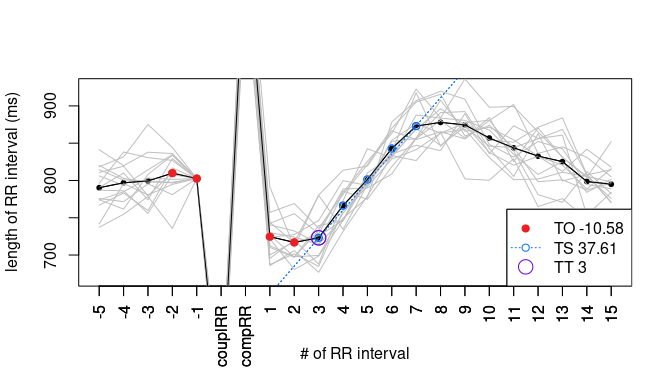Per default the VPCS is zoomed in. If you want to also see the CPI and CMI use cropped = FALSE.

plot(hrtl, cropped = FALSE) # shows also coupling and compensatory interval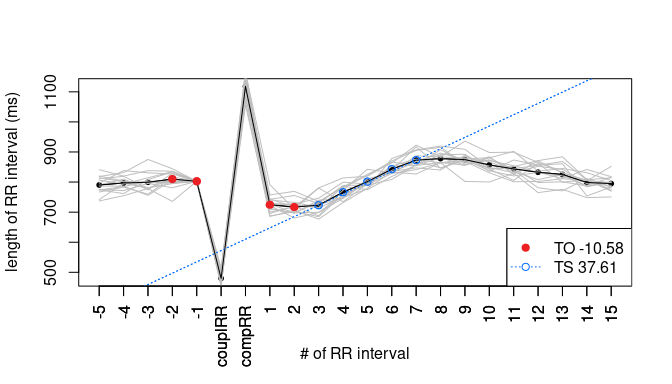## Implementation and Functions

### HRT Object

#### Slots

An HRT object saves the data of one VPCS and its HRT results. It consists of the following slots:

• Intervals
• preRRs: preceding regular intervals, 5 per default
• couplRR: CPI
• compRR: CMI
• postRRs: following regular intervals, 15 per default
• HRT Parameters
• TO
• TS
• TT
• nTS: normalised TS, for more Information see chapter Normalisation of Turbulence Slope
• Line coefficients
• intercept: The intercept of the TS regression line that is needed for plotting
• nintercept: Analogously, the intercept of nTS

#### Functions

• getRRs    This function returns all intervals saved in the HRT object. This if helpful for i.e. calculating an averaged VPCS out of a set of HRT objects.

• plot    Per default plot displays a zoomed in plot of the VPCS with highlighted TO and TS and a legend given the rounded HRT parameter values. In addition to the common parameters of graphics.plot the method accepts the following parameters:
• cropped: switches between showing a zoomed in version and the full VPCS including CPI and CMI, the default is TRUE
• add: adds the plot to the current one
• TT: highlights TT and includes it to the legend
• paramsLegend: switches between showing and hiding the parameter values in the legend, the default is TRUE
• colTO, colTS and colTT determine the colour in which the different parameters are highlighted in the plot (red, blue and purple per default).

### HRTList Object

An HRTList object is created when vectorToHRT is called. All HRT parameters are calculated automatically in this process and can be called with the getHRTParams-methods.

#### Slots

The HRTList object sums up all HRTs found in the given dataset. The slots are:

• name: The name of the object if given to vectorToHRT
• IL: the average length of all intervals in the given cleaned vector (see VectorToHRT: Cleaning Input for more information)
• pos: the indices of the CPIs as found in the given vector
• HRTs: list of all HRT objects found
• avHRT: an avHRT object averaged from all HRTs
• RMSSD: the HRV parameter RMSSD calculated from all intervals in the given cleaned vector (see VectorToHRT: Cleaning Input for more information)

#### Functions

• calcAvHRT    The function calculates the parameters of the averaged HRT. This is called automatically when using vectorToHRT with default parameters. If the avHRT should be calculated differently the following options are available:
• av: The function with which averaging should be done: mean or median.
• orTO and orTS: sets the order in which TO and TS should be calculated. With avAfter the parameter is assessed separately for every HRT and averaged afterwards, with avBefore the intervals of all VPCSs are averaged first and the parameter is assessed afterwards. The default is avAfter for orTO and avBefore for orTS.
• IL: the average interval length that is needed to calculate nTS. The default value is automatically calculated from the whole cleaned vector (see VectorToHRT: Cleaning Input for more information) when calling vectorToHRT and saved in the IL slot of the HRTList object.
• normIL: the interval length to which the other parameters should be normalised. The default is 800 ms.
• normHallstrom: Should nTS be normalised with the method by Hallstrom et al. or just based on the interval length? The default is TRUE.
• coTO, coTS and coTT: The cut-off that should be used to calculate the reliability check for the different parameters. The default is coTO 0, coTS 2.5 and coTT 10.
• getResults    This function returns either the HRT class or the parameter values. You can determine the output with
• type: “class” returns the HRT class (system HRT0-2 or HRTA-C depending on TT), “parameter” returns the HRT parameters and “full” returns the HRT parameters and the p-values of the reliability check.
• TT: Should TT be included in the return? The default is TRUE.
• nTS: Switches between giving TS (default) and nTS.
• safe: Per default safe is TRUE so only results that are reliable are returned. For not reliable results the function returns “NR” or, if num is TRUE, NA.
• pmax: The cut-off of the p-value to determine reliability. Per default this is 0.05.
• num: Forces the function to return numerics. Keep in mind that this is inapplicable when using type “class”, in this case the function gives a warning and returns NA. With type “full” num is ignored, because in that case the result is already numeric.
• coTO, coTS and coTT: Analogously to calcAvHRT the cut-off that should be used to determine whether the parameter values are normal. The default is coTO 0, coTS 2.5 and coTT 10. Be sure to give the same cut-offs to calcAvHRT and getResults if you don’t use the result, otherwise the p-values won’t match the results.
• getHRTParams    Returns the values of the given slot of all HRT objects in the HRTList. This can be used to quickly list all separate HRT parameters of an HRTList. Although the function name focuses on the HRT parameters, it can return any other slot of the HRT objects.
• getPositions    Returns the positions of the couplRRs which is identical to HRTList@pos.
• plot    Analogously to the plot function of the HRT object HRTList objects can be plotted. This function plots the avHRT and adds the VPCSs of all HRTs as grey lines in the background.

### avHRT Object

An avHRT object is stored in an HRTList and inherits from the HRT object. It is averaged from the other HRTs in the HRTList automatically and can be recalculated with calcAvHRT.

#### Slots

In addition to the HRT slots avHRT stores data about its calculation and the parameter validity:

• av, orTO and orTS: for more information see HRTList: Functions (calcAvHRT)
• pTO, pTS, pTT and pnTS: p-values from the reliability check, for more information see Reliability Check
• nRMSSD: the RMSSD normalised to the a given heart rate, per default to 75 bpm

### vectorToHRT

This is the core function of the package. It finds VPCs, checks the respective VPCS for validity and saves the results in an HRTList object. Its parameters are

• input: RR interval data that should be searched for HRT. Data formatted as timestamps should be converted before using vectorToHRT.
• annotations: If no annotations are given vectorToHRT searches for matching patterns of interval lengths in the given vector regardless of any other information in respect to the type of beats. Therefore, the function could also save HRTs based on atrial premature complexes or other arrhythmia if the surrounding intervals match the filter rules (for more information see Methods & Background: Filter Rules). If annotations are given the function only checks the intervals marked to stem from ventricular beats. This leads to more accurate results and speeds up the runtime considerably. The annotations should match the beats at the end of the intervals.
• PVCAnn: A character or string with which the VPCs are marked in the annotation vector. The default is “V”.
• normIL: The interval length to which the other parameters should be normalised. The default is 800 ms.
• normHallstrom: Should nTS be normalised with the method by Hallstrom et al. or just based on the interval length? The default is TRUE.
• numPreRRs and numPostRRs: The number of regular intervals before and after the CPI and CMI, respectively, on which the filter rules are applied and from which TS and nTS are being calculated. The default is 5 and 15, respectively.
• inputName: You can give a name to the HRTList to easier organise your data. If no name is given, the slot is set with NA.
• minHRT: This sets the minimal amount of HRTs that have to be found. Per default an HRTList is only created if the vector contains 5 or more HRTs.
• cleaning: To calculate IL and RMSSD the data is cleaned per default. (for more information see VectorToHRT: Cleaning Input).

#### Cleaning Input

The IL and RMSSD can be highly biased through outliers. Since ECG data can include artefacts, especially at the end and the beginning, RHRT cleans the data before calculating these parameters. Intervals are removed if they

• are greater than 2000 or less than 300 ms or
• differ more than 20 % of their own value from the next interval.

## Methods & Background

### Filter Rules

To ensure snippets free of any bias and containing effective VPCs, the VPCSs are filtered based on their interval lengths. The first publication to mention filter rules was Grimm et al.. With little variations these are the criteria that are used in the package as possible VPCSs are only saved as HRT objects if they match the following criteria:

1. Filter rules for CPI and CMI:

• CPI must have a maximal length of 80 %
• CMI must have a minimal length of 120 %

Both intervals are compared to the reference interval (RFI). This interval is calculated as the mean of the preceding intervals before the coupling interval.

2. Filter rules for regular intervals:

• The length has to be between 300 ms and 2000 ms
• They must not differ more than 20 % from RFI
• or more than 200 ms from the preceding interval

How many preceding and following intervals of CPI and CMI are checked is based on numPreRRs and numPostRRs of vectorToHRT. The default is 5 and 15, respectively. If any of the intervals do not fit the rules, the complete set is neglected.

### Normalisation of Turbulence Slope

HRT is influenced by the heart rate. While there is no clear conclusion for TO, TS values clearly positively correlate with the RR interval length (reviewed in Blesius et al. 2020). Therefore, RHRT calculates nTS that is normalised to a fixed interval length (800 ms per default) in addition to the common TS.

Beside the heart rate, TS is biased by the number of HRTs used to calculate it (reviewed in Blesius et al. 2020). While physiological reasons were suggested for this phenomenon (Cygankiewicz et al. 2004 and Chen 2009), Hallstrom et al. 2004 reasoned it to be a mathematically induced relation based on the number of VPCSs as well as the number of postRRs to determine TS. They proposed a method to normalise TS in which, firstly, TS is normalised to a HR of 75 bpm (which is 800 ms interval length). Here, it makes no mathematical difference whether TS is normalised or the intervals themselves before assessing TS. Secondly, the following formula is used:

nTS = TS - ( 0.02475 * (numPostRRs-2)^0.9449 * (RMSSD / √#VPCSs) )

RHRT uses this normalisation per default. This can be changed with the boolean parameter normHallstrom in vectorToHRT and calcAvHRT.

### Reliability Check

The HRT parameter values pre se do not give any information about 1) how many VPCSs have been used to determine them and 2) how reliable the values are. However, two identical values are inherently different if one is calculated from a VPCS with a highly varying values and the other from a high amount of VPCS with hardly any variation. Still, HRT classification generally does not take this into account.

RHRT implements a reliability check to give the opportunity to only use HRT parameter values that are reliable to a desired extent. This check consists of a one-sided t-test (t.test of the stats package) off all separate values against the respective cut-off of the parameter. The resulting p-value implicates the possibility of the classification being true based on being the combination of average and variability of the parameter values and therefore the reliability of the averaged value.

These t-tests are being done automatically during calcAvHRT which is called by vectorToHRT. The default values of the cut-offs are 0 for TO, 2.5 for TS as well as nTS and 10 for TT. getResults returns the results if reliable. However, it returns all results ignoring the reliability check via the boolean parameter safe and changes the p-value cut-off with pmax (0.05 per default).

Keep in mind that the parameter value cut-offs coTO, coTS and coTT are only used to compare the values and classify them. They are not related to the identically named parameters of calcAvHRT that are used for the t-tests.

### Calculation Order

The order in which the HRT parameters are calculated has an impact on the resulting values (Chen 2011). Though Schmidt et al. 1999 proposed to first calculate an averaged tachogram and determine TS then and for TO to first assess it from the separate VPCSs and average the results afterwards, the order gets switched in some studies as reviewed in Blesius et al. 2020. Therefore, RHRT gives the opportunity to change the calculation order for TO and TS through the parameters orTO and orTS of calcAvHRT. By default the order is as suggested by Schmidt et al. Additionally with av you can switch between mean and median as averaging function.

## Example Pipelines

### Determining the HRT class of a person

The main focus of the package is to determine the HRT parameters or class of a person by a long-term ECG measurement. Load the data as a numeric vector and use vectorToHRT to find HRTs, then getResults and plot to check the results:

hrtl <- vectorToHRT(testdataLong) # create the HRTList
getResults(hrtl) # get the averaged HRT parameters
##  "HRT0"
plot(hrtl) # plot the HRTs and check the variability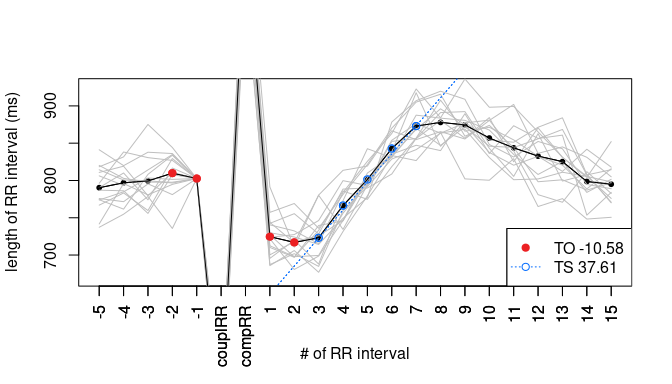The results do not pass the reliability check so we get “NR” as HRT class. The plot shows that firstly TO is near to zero and secondly there is a high variability in the VPCSs. We can go deeper into the data by checking the exact parameters (including TT as an additional hint to the person’s status) and zooming out of the plot:

round(
getResults(hrtl, "full", TT = TRUE),
digits = 2) # get the parameters and p-values of the variability check
##     TO     TS     TT    pTO    pTS    pTT
## -10.58  37.61   3.00   0.00   0.00   0.00
plot(hrtl, cropped = FALSE) # plot the full VPCSsAs expected TO is not reliable with a p-value over 0.05. The VPCSs still seem to fluctuate a lot with the first two postRRs that determine TO varying above and below the ones of the avHRT. We can can get a picture on all TO values by using getHRTParams:

tos <- getHRTParams(hrtl, "TO")
tos
##   -12.264829  -6.349883 -11.856998  -9.011983 -13.733660 -10.859878
##   -10.357064 -13.174994 -12.743754  -8.427299 -11.495815  -8.892588
##  -11.795365  -6.502960 -11.165541
summary(tos)
##    Min. 1st Qu.  Median    Mean 3rd Qu.    Max.
## -13.734 -12.061 -11.166 -10.576  -8.952  -6.350
boxplot(tos)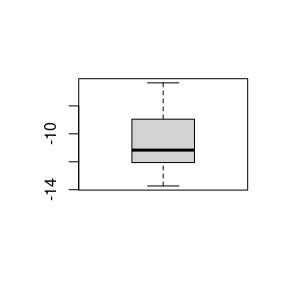These results can help to come to a well-founded decision on whether to classify the patient as HRT0/HRTA and trust the TO value or rather classify them conservatively as HRT1/HRTB.

### Comparing HRT results with different methodological parameters

This is an example how the package can be used to analyse the HRT methodology. Due to data size we use a set of HRTs of one person rather than a set of averaged HRTs. For example, we can compare the difference in TS values when using different numPostRRs.

hrtl10 <- getHRTParams(vectorToHRT(testdataLong, numPostRRs = 10), "TS")
hrtl20 <- getHRTParams(vectorToHRT(testdataLong, numPostRRs = 20), "TS")
boxplot(hrtl10, hrtl20, names = c("TSRR = 10", "TSRR = 20"))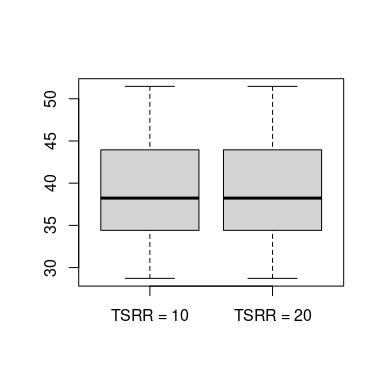t.test(hrtl10, hrtl20)
##
##  Welch Two Sample t-test
##
## data:  hrtl10 and hrtl20
## t = 0, df = 28, p-value = 1
## alternative hypothesis: true difference in means is not equal to 0
## 95 percent confidence interval:
##  -5.116392  5.116392
## sample estimates:
## mean of x mean of y
##  39.36397  39.36397